# Test: Matrices And Determinants (Competition Level)

## 20 Questions MCQ Test Mathematics (Maths) Class 12 | Test: Matrices And Determinants (Competition Level)

Description
This mock test of Test: Matrices And Determinants (Competition Level) for JEE helps you for every JEE entrance exam. This contains 20 Multiple Choice Questions for JEE Test: Matrices And Determinants (Competition Level) (mcq) to study with solutions a complete question bank. The solved questions answers in this Test: Matrices And Determinants (Competition Level) quiz give you a good mix of easy questions and tough questions. JEE students definitely take this Test: Matrices And Determinants (Competition Level) exercise for a better result in the exam. You can find other Test: Matrices And Determinants (Competition Level) extra questions, long questions & short questions for JEE on EduRev as well by searching above.
QUESTION: 1

### If B is a non–singular matrix and A is a square matrix, then det (B–1 AB) is equal to

Solution:

A is a square matrix and B is non-singular matrix, so B is invertible and a square matrix.

det(B−1 AB)

=det(B−1 BA)

=det(InA)  (where In is an identity matrix of order n )

=det(A)

QUESTION: 2

### If the system of equations x + 2y + 3z = 4, x + λy + 2z = 3, x + 4y + μz = 3 has an infinite anumber of solutions then

Solution:

Explanation : x+2y+3z=4

x+py+2z=3

x+4y+z=3

x = Δx/Δ, y = Δy/Δ, z = Δz/Δ

Δ = 0, Δx = Δy = Δz = 0

As we know that Δy = 0

A = {(1,4,3) (1,3,2) (1,3,μ)} = 0

3μ + 9 + 8 - 9 - 6 - 4μ = 0

=> μ = 2

As Δz = 0

= {(1,2,4) (1,p,3) (1,4,3)} = 0

=> 3p + 16 - 6 - 4p - 12 -6 = 0

=> p = 4

QUESTION: 3

### If a, b, c are non zeros, then the system of equations (α + a) x + αy + αz = 0 αx + (α + b)y + αz = 0  αx + αy + (α + c) z = 0 has a non–trivial solution if

Solution:

For non trival solution, D = 0
{(α + a, α, α)(α, α + b, α)(α, α, α + c)} = 0
R1→ R1 - R2,  R2 → R2 - R3
{(a, -b ,0)(0, b ,-c)(α, α, α + c)}
abα + abc + acα + bcα = 0
⇒ abc = −α(ab + bc + ca)
⇒ 1/α = −(1/c+ 1 / a + 1 / b)
α−1 = −(a−1+b−1+c−1)

QUESTION: 4

Let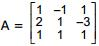and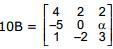. If B is the inverse of matrix A, then α is

Solution:

Since, B is the inverse of matrix A.
So, 10A−1 = {(4,2,2) (-5,0,α) (1,-2,3)}
⇒ 10A-1 . A = {(4,2,2) (-5,0,α) (1,-2,3)} . {(1,-1,1) (2,1,-3) (1,1,1)}
⇒ 10I = {(10,0,0) (-5+α, 5+α, -5+α) (0,0,10)}
⇒ {(10,0,0) (0,10,0) (0,0,10)} = {(10,0,0) (-5+α, 5+α, -5+α) (0,0,10)}
−5+α=0
⇒ α=5

QUESTION: 5

The value of a for which system of equations,

a3x + (a + 1)3 y + (a + 2)3z = 0,
ax + (a + 1) y + (a + 2) z = 0,
x + y + z = 0, has a non–zero solution is

Solution:
QUESTION: 6

If ω is a cube root of unity and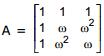, then A–1 equal to

Solution:
QUESTION: 7

Let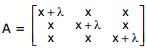, then A-1 exists if

Solution:
QUESTION: 8

Let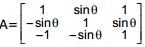where 0 ≤ θ < 2π, then

Solution:
QUESTION: 9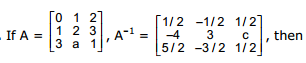Solution:
QUESTION: 10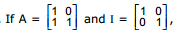then which one of the following holds for all n ≥ 1, by the principle of mathematical induction ?

Solution:
QUESTION: 11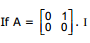is the unit matrix of order 2 and a, b are arbitrary constants, then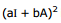is equal to

Solution:
QUESTION: 12

Identity the correct statement(s)

Solution:
QUESTION: 13

If a, b, c > 0 & x, y, z ∈ R then the determinant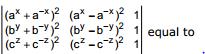Solution:
QUESTION: 14

IF x, y , z ∈ R Δ =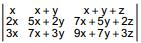= -16 then value of x is

Solution:
QUESTION: 15

The determinant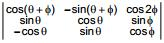is

Solution:
QUESTION: 16

If A, B, C are angles of a triangle ABC, then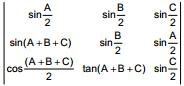is less than or equal to

Solution:

Solution :- sin(A+B+C) = cos(A+B+C)/2 = 0

Hence, sinA/2 sinB/2 sinC/2 ≤ 1/8

QUESTION: 17

Let f(x) =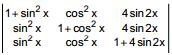then the maximum value of f(x) is

Solution:
QUESTION: 18

Value of the D =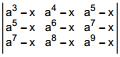is

Solution:
QUESTION: 19

If f(x) =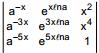,  then

Solution:

f(x) ={(a-x, ex/na, x^2) (a-3x, e3x/na, x4) (a-5x, e5x/na, 1)}
f(-x) = - {(a-x, ex/na, x2) (a-3x, e3x/na, x4) (a-5x, e^5x/na, 1)}
Adding (1) and (2) we get
f(x) + f(-x) = 0

QUESTION: 20

D =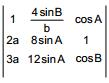is (where a, b, c are the sides opposite to angles A, B, C respectively in a triangle)

Solution:

a/sinA = b/sinB = c/sinC = 2R
A = 2RsinA,   b = 2RsinB,   c = 2RsinC
{(1, 4sinB/b, cosA) (2a, 8sinA, 1) (3a, 12sinA, cosB)}
= {(1, 4sinB/(2RsinB), cosA) (4RsinA, BsinA, 1) (6RsinA, 12sinA, cosB)}
= 1(8sinAcosB- 12sinA) - 2/R(4RsinAcosB - 6RsinA) + cosA(48Rsin^2A - 48Rsin^2A)
= 8sinAcosB - 12sinA -8sinAcosB + 12sinA
= 0